# t-SNE算法

t-SNE(t-distributed stochastic neighbor embedding)是用于降维的一种机器学习算法，是由 Laurens van der Maaten 和 Geoffrey Hinton在 08 年提出来。t-SNE 是一种非线性降维算法，非常适用于高维数据降维到 2 维或者 3 维，进行可视化。在实际应用中，t-SNE很少用于降维，主要用于可视化，可能的原因有以下几方面：

• 当我们发现数据需要降维时，一般是特征间存在高度的线性相关性，此时我们一般使用线性降维算法，比如PCA。即使是特征之间存在非线性相关，也不会先使用非线性降维算法降维之后再搭配一个线性的模型，而是直接使用非线性模型；
• 一般 t-SNE 都将数据降到 2 维或者 3 维进行可视化，但是数据降维降的维度一般会大一些，比如需要降到 20 维，t-SNE 算法使用自由度为 1 的 t 分布很难做到好的效果，而关于如何选择自由度的问题，目前没有研究；
• t-SNE 算法的计算复杂度很高，另外它的目标函数非凸，可能会得到局部最优解；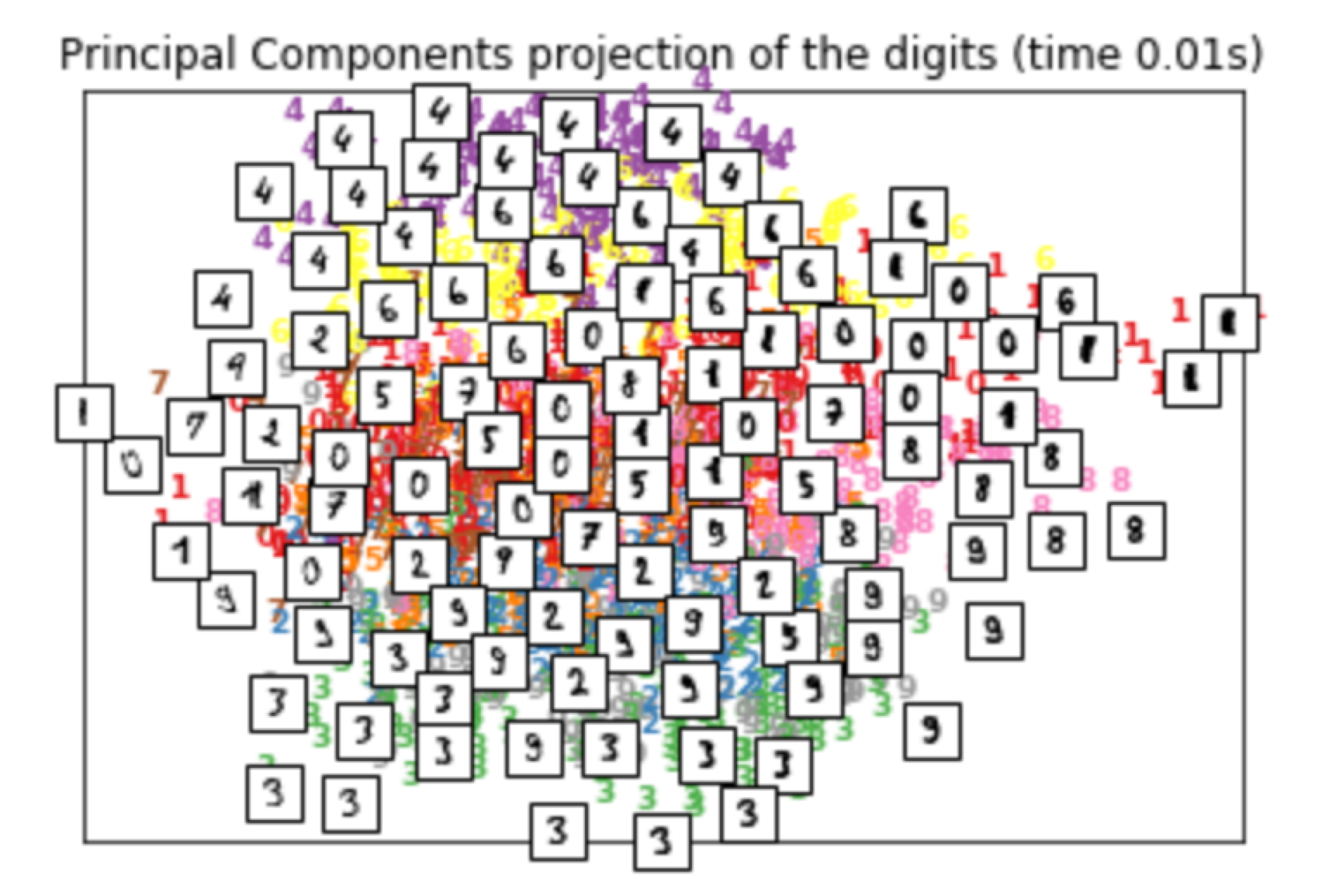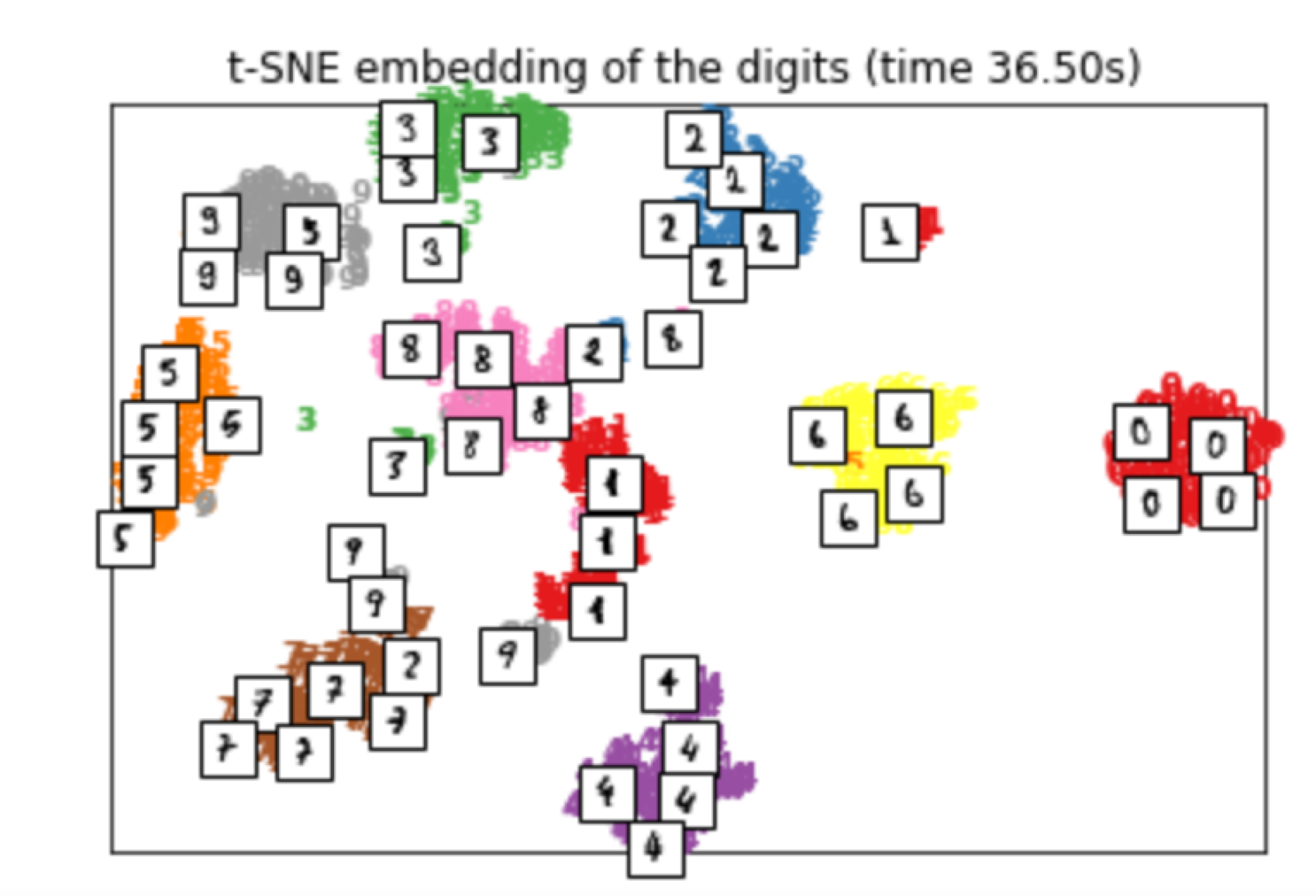## 一、SNE

1、基本思想

t-SNE算法由 SNE 改进而来，所以先来介绍 SNE。给定 n 个高维数据 x1,x2,...,xn x 1 , x 2 , . . . , x n ${{x}_{1}},{{x}_{2}},...,{{x}_{n}}$，若将其降维至 2 维，SNE 的基本思想是若两个数据在高维空间中是相似的，那么降维至 2 维空间时它们应该离得很近

2、相似性计算

SNE使用条件概率来描述两个数据之间的相似性，假设 xi,xj x i , x j ${{x}_{i}},{{x}_{j}}$是高维空间中的两个点，那么以点 xi x i ${{x}_{i}}$为中心构建方差为 σi σ i ${{\sigma }_{i}}$的高斯分布，使用 pj|i p j | i ${{p}_{j|i}}$表示 xj x j ${{x}_{j}}$ xi x i ${{x}_{i}}$邻域的概率，如果 xj x j ${{x}_{j}}$ xi x i ${{x}_{i}}$很近，那么 pj|i p j | i ${{p}_{j|i}}$很大，反之， pj|i p j | i ${{p}_{j|i}}$很小， pj|i p j | i ${{p}_{j|i}}$定义如下：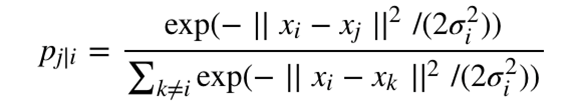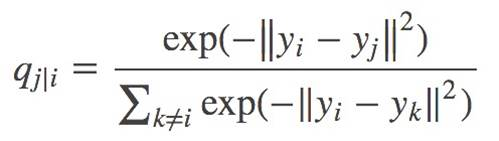3、目标函数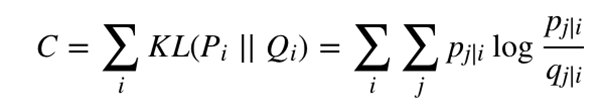• pj|i p j | i ${{p}_{j|i}}$ = 0.9，也就是 xj x j ${{x}_{j}}$ xi x i ${{x}_{i}}$很近时，如果算法计算映射到低维空间的 pj|i p j | i ${{p}_{j|i}}$ = 0.1，也就是映射后 xj x j ${{x}_{j}}$ xi x i ${{x}_{i}}$很远，那么此时目标函数会有一个很大的损失。SNE算法也因此保证了数据的局部性；
• pj|i p j | i ${{p}_{j|i}}$ = 0.1，也就是 xj x j ${{x}_{j}}$ xi x i ${{x}_{i}}$很远时，如果算法计算映射到低维空间的 pj|i p j | i ${{p}_{j|i}}$ = 0.9，也就是映射后 xj x j ${{x}_{j}}$ xi x i ${{x}_{i}}$很近，那么此时目标函数会有一个很小的损失，实际上我们并不希望得到这样的结果，一个大的损失才是我们想要的，但由于 K- L 散度本身的不对称性，这一缺陷无法避免，后续我们看 t-SNE 算法如何对其进行改进。

4、SNE缺点

• 不对称导致梯度计算复杂，对目标函数计算梯度如下，由于条件概率 pj|i p j | i ${{p}_{j|i}}$ 不等于 pi|j p i | j ${{p}_{i|j}}$ qj|i q j | i ${{q}_{j|i}}$ 不等于 qi|j q i | j ${{q}_{i|j}}$，因此梯度计算中需要的计算量较大。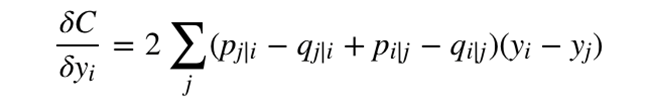• 拥挤问题。所谓拥挤问题，顾名思义，就是不同类别的簇挤在一起，无法区分开来，这就是拥挤问题。有的同学说，是不是因为SNE更关注局部结构，而忽略了全局结构造成的？实际上，拥挤问题的出现与某个特定算法无关，而是由于高维空间距离分布和低维空间距离分布的差异造成的。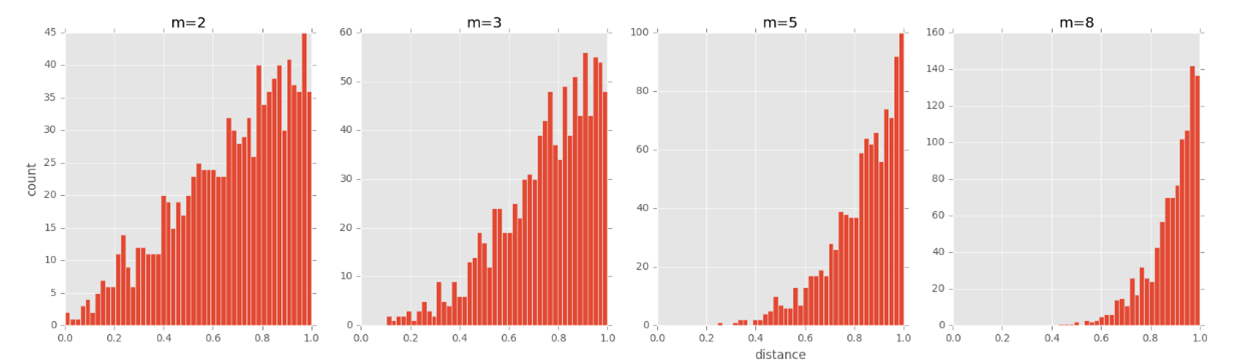• 由于K - L散度本身的不对称性，使得SNE算法只关注数据局部性而忽略了数据的全局性。

## 二、t-SNE

1、对称SNE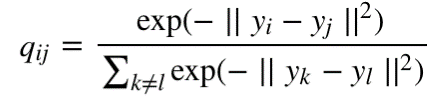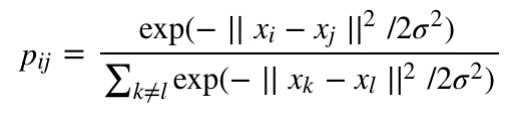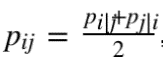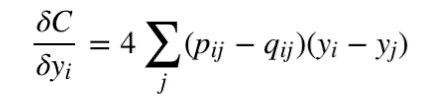2、引入 t 分布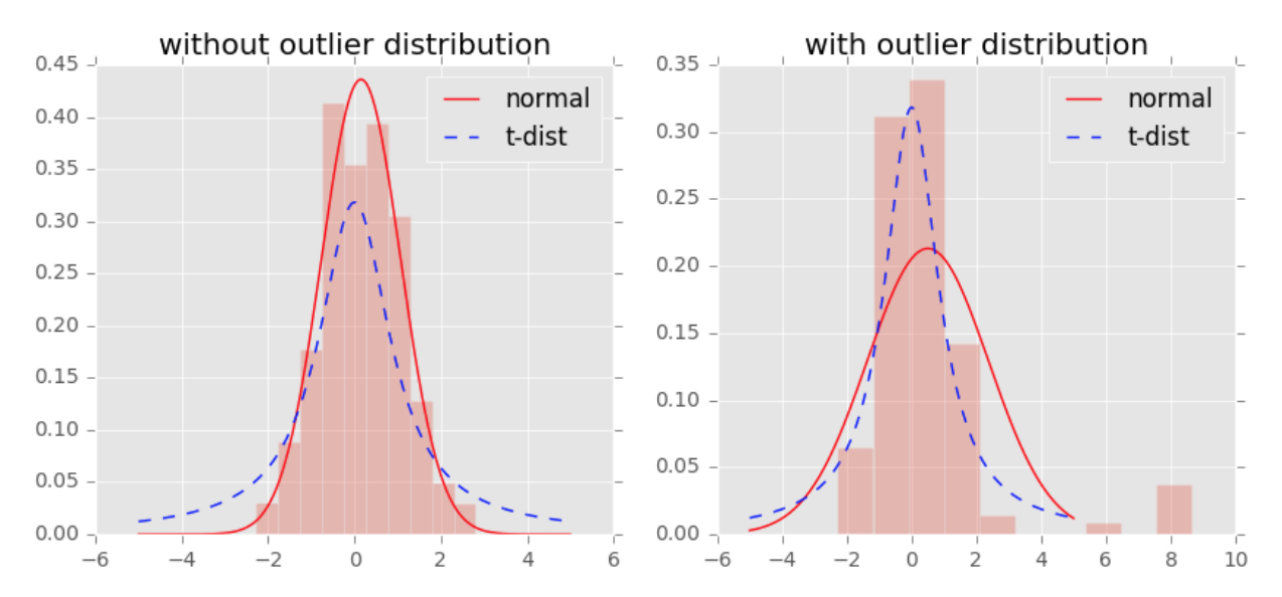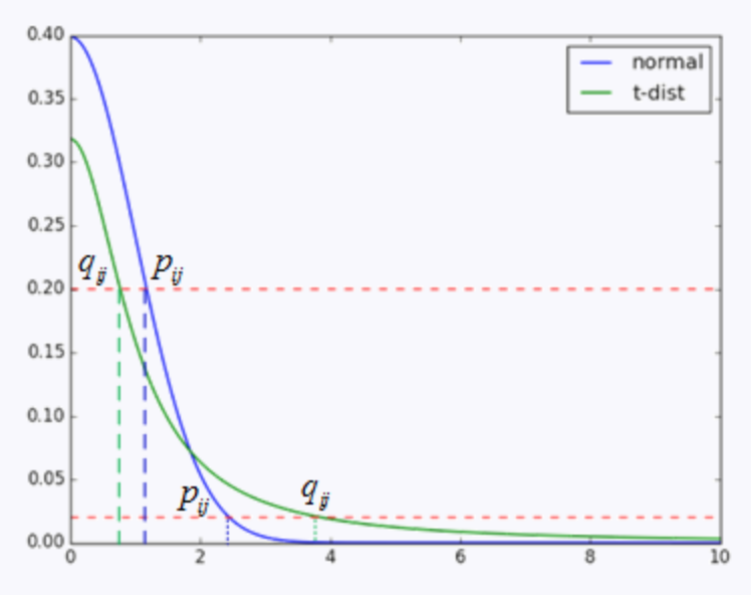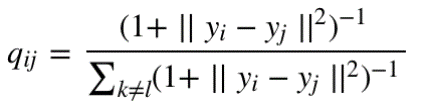3、总结

• 把SNE变为对称SNE，提高了计算效率，效果稍有提升
• 在低维空间中采用了t分布代替原来的高斯分布，解决了拥挤问题，优化了SNE过于关注局部特征忽略全局特征的问题
下面是 SNE 算法和 T-SNE 算法的可视化结果对比，可以看到， T-SNE 算法的效果要远远好于 SNE。

SNE：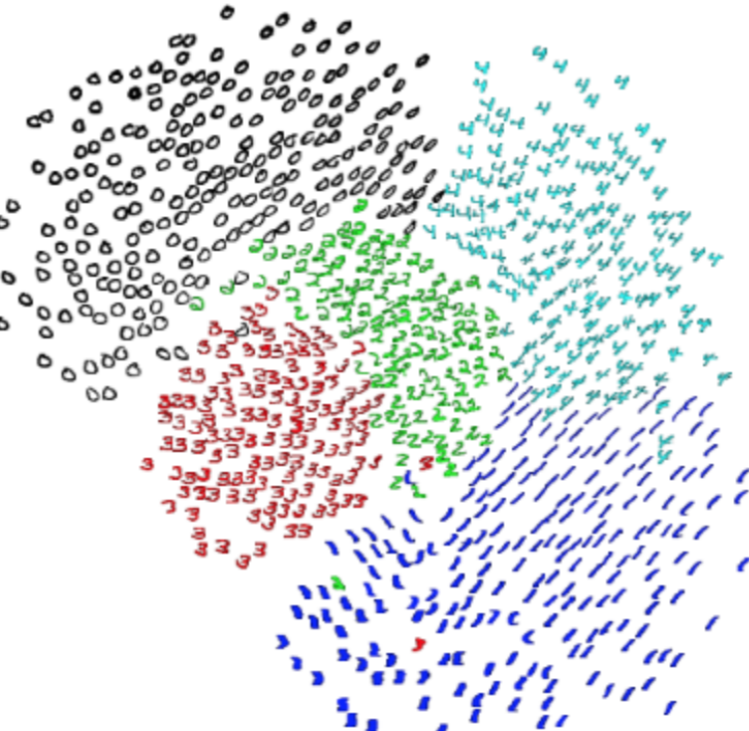T-SNE：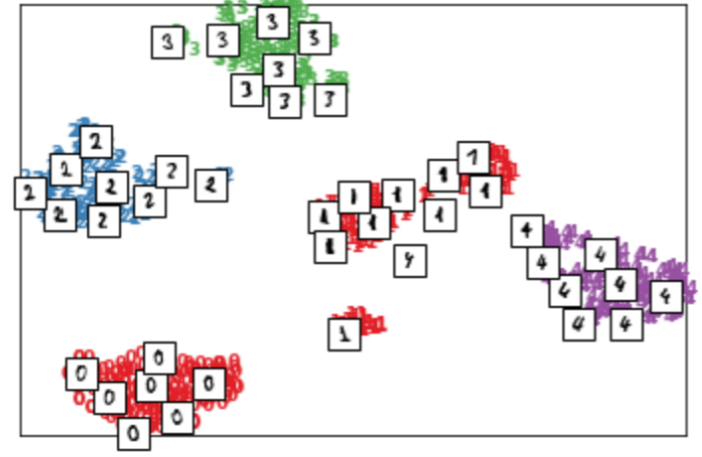• 主要用于可视化，很难用于其他目的.比如降维到10维，因为t分布偏重长尾，1个自由度的 t 分布很难保存好局部特征，可能需要设置成更高的自由度；
• t-SNE 没有唯一最优解，且不能用于预测，比如测试集合降维，因为他没有显式的预估部分，不能在测试集合直接降维；
• 速度慢
01-306204
04-13677604-301万+
04-161万+
01-225099
06-131万+
03-111万+
09-19
09-308万+
11-281304